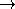## CGAL::min_max_element

### Definition

The function min_max_element computes the minimal and the maximal element of a range. It is modeled after the STL functions min_element and max_element. The advantage of min_max_element compared to calling both STL functions is that one only iterates once over the sequence. This is more efficient especially for large and/or complex sequences.

#include <CGAL/algorithm.h>

template < class ForwardIterator >
std::pair< ForwardIterator, ForwardIterator >
 min_max_element ( ForwardIterator first, ForwardIterator last)
returns a pair of iterators where the first component refers to the minimal and the second component refers to the maximal element in the range [first, last). The ordering is defined by operator< on the value type of ForwardIterator.

template < class ForwardIterator, class CompareMin, class CompareMax >
std::pair< ForwardIterator, ForwardIterator >
 min_max_element ( ForwardIterator first, ForwardIterator last, CompareMin comp_min, CompareMax comp_max)
returns a pair of iterators where the first component refers to the minimal and the second component refers to the maximal element in the range [first, last).
Requirement: CompareMin and CompareMax are adaptable binary function objects: VT  ×  VTbool where VT is the value type of ForwardIterator.

### Example

The following example program computes the minimal and maximal element of the sequence (3,6,5). Hence the output is min = 3, max = 6.

```#include <CGAL/algorithm.h>
#include <vector>
#include <iostream>

using std::vector;
using std::pair;
using std::cout;
using std::endl;
using CGAL::min_max_element;

int main()
{
vector< int > v;
v.push_back(3);
v.push_back(6);
v.push_back(5);
typedef std::vector< int >::iterator iterator;
pair< iterator, iterator > p = min_max_element(v.begin(), v.end());
cout << "min = " << *p.first << ", max = " << *p.second << endl;
return 0;
}
```